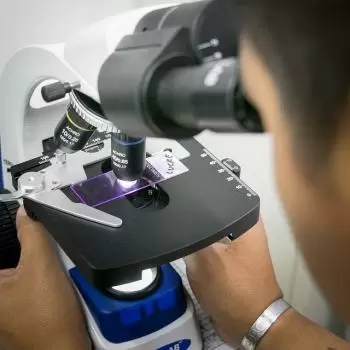# The Scientific Method: Steps, Characteristics and ExamplesThe scientific method is the way in which the scientific community proceeds to develop verifiable scientific laws in reality through reliable knowledge. This method consists of a series of stages without the influence of the subjectivity of the scientist carrying out the research.

The main characteristics of the scientific method consist of:

1. Making a hypothesis about the object of study.

2. Data collection from an empirical point of view.

3. Analyze the results of the data obtained rigorously.

The paternity of the scientific method is attributed to Galileo Galilei in the 17th century. Hence also the name Galilean method.

## What Is the Scientific Method?

The scientific method is a set of steps used to investigate phenomena and solve problems. It is characterized by being a logical, systematic and iterative process that allows reliable conclusions to be drawn from the analysis of evidence.

The steps of the scientific method are usually summarized in the following stages: formulation of hypotheses, data collection, data analysis, interpretation of results and drawing conclusions.

Although these stages are presented in a linear order, in practice there is usually a great deal of interaction between them.

## What Is the Scientific Method For?

The scientific method is a systematic way of solving problems or answering questions by using logic and empirical evidence. Simply put, the scientific method is a process for making discoveries or solving problems in an organized and methodical way.

The scientific method is a systematic form of investigation that seeks to find answers to questions about the natural sciences, observations made in everyday life.

## Steps of the Scientific Method

The different stages of the scientific method are detailed below:

1. Formulation of a problem: The first step in applying the scientific method is to formulate a problem or question to be investigated. The question asked may be triggered by natural curiosity or by the desire to solve a practical problem.

2. Data collection: Once the problem has been formulated, it is necessary to collect relevant data to try to answer the question posed. Data can be obtained through direct or indirect observation, literature research, or experiment.

3. Build a model: Once the data has been gathered, it is necessary to build a model to try to explain the results obtained. This involves developing hypotheses and theories that describe the problem at hand.

4. Testing: Models must be tested to verify their validity and accuracy. These tests can be performed through controlled experiments or statistical analysis.

5. Analysis of the results: The last step of the scientific method involves analyzing the results obtained and evaluating whether the hypotheses previously made are confirmed or refuted. This makes it possible to identify the conclusions reached on the topic studied.

## Types of Scientific Methods

Below are the different types of scientific methods:

1. Inductive method: draws general conclusions from a set of data or particular facts. For example, if we observe that all the cats we have seen have hair, we can deduce that all cats have hair.

2. Deductive method: generates particular conclusions from a general premise. For example, if we know that all cats have hair, we can deduce that the cat we just saw also has hair.

3. Experimental method: establishes relationships between variables, and is based on the manipulation of one or more elements. For example, if we want to see if a certain medication improves physical performance, we will carry out a controlled trial where some subjects take the medication and others do not.

4. Statistical method: This method is used to analyze quantitative data and draw general conclusions from them. For example, if we collect information on the educational level of the inhabitants of a certain city, we can use statistical techniques to draw conclusions about the average level of schooling in that area.

## Scientific Method Example

Most of the laws of physics have been derived from the scientific method. Some examples are the discovery of radioactivity by Antoine Henri Becquerel or Archimedes' principle that explains why hot air balloons can fly.

Below we develop a scientific method to prove or reject the hypothesis of Archimedes' principle:

### Example of Archimedes' Principle

Archimedes' principle is a law of fluid mechanics that says that an object completely immersed in a fluid displaces a volume of liquid equal to the volume of the object itself.

Applying the previous steps we can verify the following:

1. Observation: when an object is submerged in a tank of water, we observe that the water level rises and we want to know if the difference in level depends on the volume of the object.

2. Data Collection: We collect data from different objects and liquids by calculating their volumes and the density of each liquid.

3. Build the model: we define a series of tests and tests in which we submerge the different objects in different liquids.

4. Carrying out the test: During the experiments we measured the volume of liquid displaced.

5. Analysis of results: we compare the volume of the displaced liquid and compare it with the volume of the submerged object. We observe what relationship there is between both volumes and we accept or reject the initial hypothesis.

##### What Are the Advantages of Nuclear Power Plants?
Author:

Published: March 13, 2023
Last review: March 13, 2023